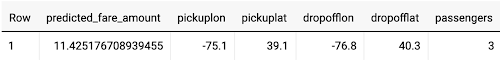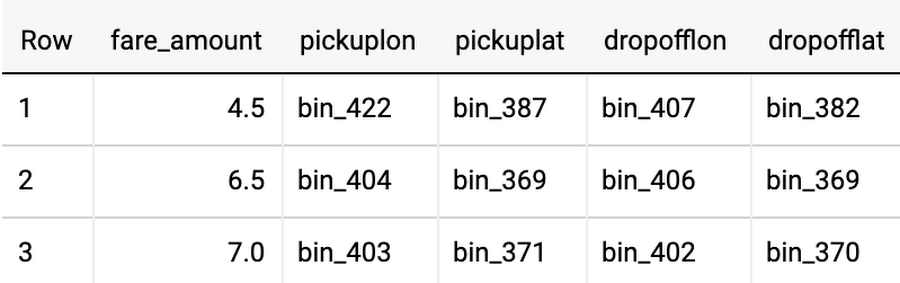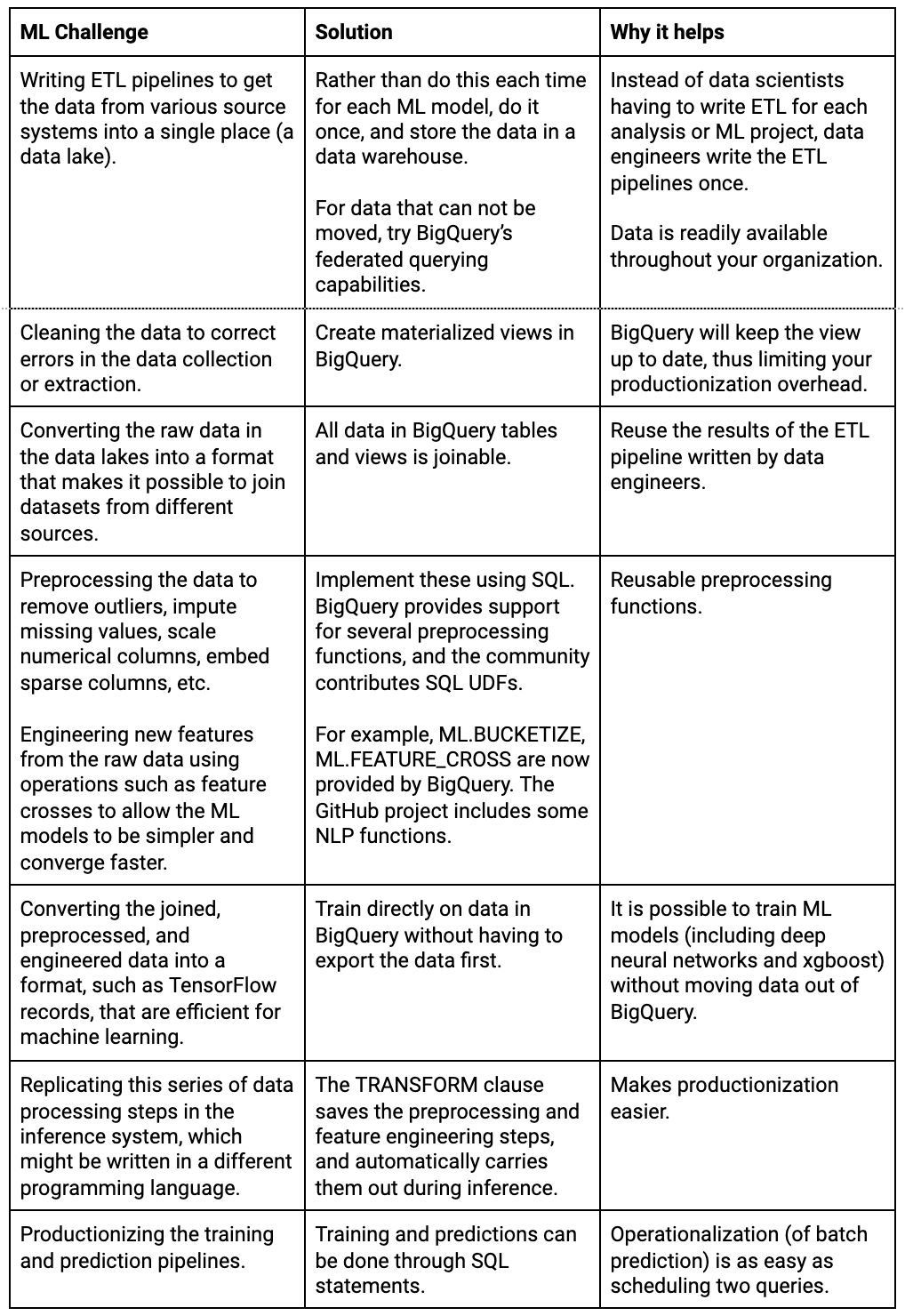# BigQuery 数据转换简化大法：让机器学习更轻松高效• 编写ETL流水线，立足单一位置（数据湖）从多种不同源系统处获取数据并注入到单一位置（数据湖）；
• 数据清洗洁，对所提取数据集合进行纠错；
• 对数据湖中的原始数据进行格式转换，将不同来源的数据纳入统一数据集；
• 数据预处理，包括清除异常值、估算缺失值、缩放数据列、嵌入稀疏列等；
• 利用特征交叉等操作在原始数据中引入新的特征工程，降低机器学习模型的复杂度并提高收敛速度；
• 将经过合并、预处理以及工程化的数据转换为TensorFlow Records等可供机器学习模型高效处理的格式；
• 在推理系统中重复整个数据处理流程，流程中各步骤可能是使用其他不同编程语言进行编写的；
• 完成训练与预测流水线的生产化转换。

### 充分发挥数据仓库中的内置机器学习优势

CREATE OR REPLACE MODEL serverlessml.model1_rawdataOPTIONS(input_label_cols=['fare_amount'], model_type='linear_reg') ASSELECT  (tolls_amount + fare_amount) AS fare_amount,  pickup_longitude AS pickuplon,  pickup_latitude AS pickuplat,  dropoff_longitude AS dropofflon,  dropoff_latitude AS dropofflat,  passenger_count*1.0 AS passengersFROM nyc-tlc.yellow.trips

### 通过计划查询推动生产化进程

SELECT * FROM ML.PREDICT(MODEL serverlessml.model1_rawdata, (SELECT   -75.1 AS pickuplon,  39.1 AS pickuplat,  -76.8 AS dropofflon,  40.3 AS dropofflat,  3 AS passengers))### BigQuery ML 中的预处理机制

SELECT  (tolls_amount + fare_amount) AS fare_amount,  pickup_longitude AS pickuplon,  pickup_latitude AS pickuplat,  dropoff_longitude AS dropofflon,  dropoff_latitude AS dropofflat,  passenger_count*1.0 AS passengersFROM nyc-tlc.yellow.tripsWHERE   trip_distance > 0  AND fare_amount >= 2.5  AND pickup_longitude > -78  AND pickup_longitude < -70  AND dropoff_longitude > -78  AND dropoff_longitude < -70  AND pickup_latitude > 37  AND pickup_latitude < 45  AND dropoff_latitude > 37  AND dropoff_latitude < 45  AND passenger_count > 0

CREATE OR REPLACE PRE-AGGREGATED VIEW serverlessml.cleaned_data ASSELECT ...

with raw_data AS (  SELECT *  FROM cloud-training-demos.serverlessml.cleaned_training_data), zero_norm AS (  SELECT     AVG(pickuplon) AS mean_pickuplon    , AVG(pickuplat) AS mean_pickuplat    , AVG(dropofflon) AS mean_dropofflon    , AVG(dropofflat) AS mean_dropofflat    , STDDEV(pickuplon) AS std_pickuplon    , STDDEV(pickuplat) AS std_pickuplat    , STDDEV(dropofflon) AS std_dropofflon    , STDDEV(dropofflat) AS std_dropofflat  FROM raw_data) SELECT   fare_amount  , bqutil.fn.zeronorm(pickuplon, mean_pickuplon, std_pickuplon) AS pickuplon  , bqutil.fn.zeronorm(pickuplat, mean_pickuplat, std_pickuplat) AS pickuplat  , bqutil.fn.zeronorm(dropofflon, mean_dropofflon, std_dropofflon) AS dropofflon  , bqutil.fn.zeronorm(dropofflat, mean_dropofflat, std_dropofflat) AS dropofflat  from raw_data, zero_norm

BigQuery以开箱即用的方式为多种常规机器学习操作提供支持，意味着用户在执行此类操作时，不再需要对数据进行单独的analysis pass。例如，以下代码将对输入进行分桶，从而计算纽约市的纬度与经度边界：

 SELECT   fare_amount  , ML.BUCKETIZE(pickuplon, GENERATE_ARRAY(-78, -70, 0.01)) AS pickuplon  , ML.BUCKETIZE(pickuplat, GENERATE_ARRAY(37, 45, 0.01)) AS pickuplat  , ML.BUCKETIZE(dropofflon, GENERATE_ARRAY(-78, -70, 0.01)) AS dropofflon  , ML.BUCKETIZE(dropofflat, GENERATE_ARRAY(37, 45, 0.01)) AS dropofflatfrom cloud-training-demos.serverlessml.cleaned_training_data### 利用 TRANSFORM 限制由训练产生的偏差

 SELECT * FROM ML.PREDICT(MODEL serverlessml.model1_rawdata, (SELECT   ML.BUCKETIZE(-75.1, GENERATE_ARRAY(-78, -70, 0.01)) AS pickuplon,  ML.BUCKETIZE(39.1, GENERATE_ARRAY(37, 45, 0.01)) AS pickuplat,  ML.BUCKETIZE(-76.8, GENERATE_ARRAY(-78, -70, 0.01)) AS dropofflon,  ML.BUCKETIZE(40.3, GENERATE_ARRAY(37, 45, 0.01)) AS dropofflat,  3 AS passengers))

 CREATE OR REPLACE MODEL serverlessml.model7_geoTRANSFORM(  fare_amount  , ST_Distance(ST_GeogPoint(pickuplon, pickuplat), ST_GeogPoint(dropofflon, dropofflat)) AS euclidean  , ML.FEATURE_CROSS(STRUCT(CAST(EXTRACT(DAYOFWEEK FROM pickup_datetime) AS STRING) AS dayofweek,                            CAST(EXTRACT(HOUR FROM pickup_datetime) AS STRING) AS hourofday), 2) AS day_hr  , CONCAT(     ML.BUCKETIZE(pickuplon, GENERATE_ARRAY(-78, -70, 0.01)),     ML.BUCKETIZE(pickuplat, GENERATE_ARRAY(37, 45, 0.01)),     ML.BUCKETIZE(dropofflon, GENERATE_ARRAY(-78, -70, 0.01)),     ML.BUCKETIZE(dropofflat, GENERATE_ARRAY(37, 45, 0.01))  ) AS pickup_and_dropoff)OPTIONS(input_label_cols=['fare_amount'], model_type='linear_reg', l2_reg=0.1, optimize_strategy='BATCH_GRADIENT_DESCENT') AS SELECT * FROM cloud-training-demos.serverlessml.feateng_training_data